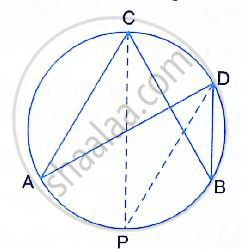Share

# In the Figure, Given Alongside, Cp Bisects Angle Acb. Show that Dp Bisects Angle Adb. - Mathematics

Course

#### Question

In the figure, given alongside, CP bisects angle ACB. Show that DP bisects angle ADB.#### Solution

Given – In the figure, CP is the bisector of ∠ABC
To prove – DP is the bisector of ∠ADB
Proof – Since CP is the bisector of ∠ACB
∴ ∠ACP = ∠BCP

But ∠ACP = ∠ADP  [Angle in the same segment

And ∠BCP = ∠BDP

But ∠ACP = ∠BCP

∴ DP is the bisector of ∠ADB

Is there an error in this question or solution?